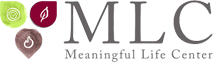The most famous equation in the world is E=mc². Einstein developed this equation in 1905, demonstrating that energy and mass are the same thing, with the incredible revelation that you can get an enormous amount of energy out of a very small amount of mass. But would you believe that the core principle of this theory was generated 3326 years ago?!

Moreover, going back to that early root over thirty centuries ago will provide us insight into the actual equation as well as its personal applications. The basic purpose of the equation is to show how much energy is contained in a given mass: E (energy) = m (the amount of mass) multiplied by c (the speed of light) squared. Simply put, all mass is really very tightly packed energy (each atom of a substance can be thought of as a little ball of concentrated energy), which can be released under certain circumstances. The energy (called kinetic energy) emerges when that mass is accelerated, and the more mass or velocity a moving object has, the greater its kinetic energy released. When you accelerate an object, the kinetic energy increases to the tune of the speed squared. For instance, if you double your speed in an automobile, the braking distance is four times longer. In other words, the braking distance is equal to the speed squared. Likewise, we can take energy (such as particles of light, called photons) and turn it into matter. Beyond the scientific implications of this equation we can derive volumes of lessons from the original E=mc² revealed thousands of years ago.

Please join Rabbi Jacobson in this groundbreaking pre-Shavuot workshop, and learn how one of (if not the) most important scientific breakthrough of our time, which set in motion many of our revolutionary technologies, is rooted in the event that took place at Sinai over three millennia ago. Discover how that event unleashed forces that would allow us to transform our material mass-filled lives into enormous forces of positive energy.

Subscribe
Notify of
Load MoreLoad More